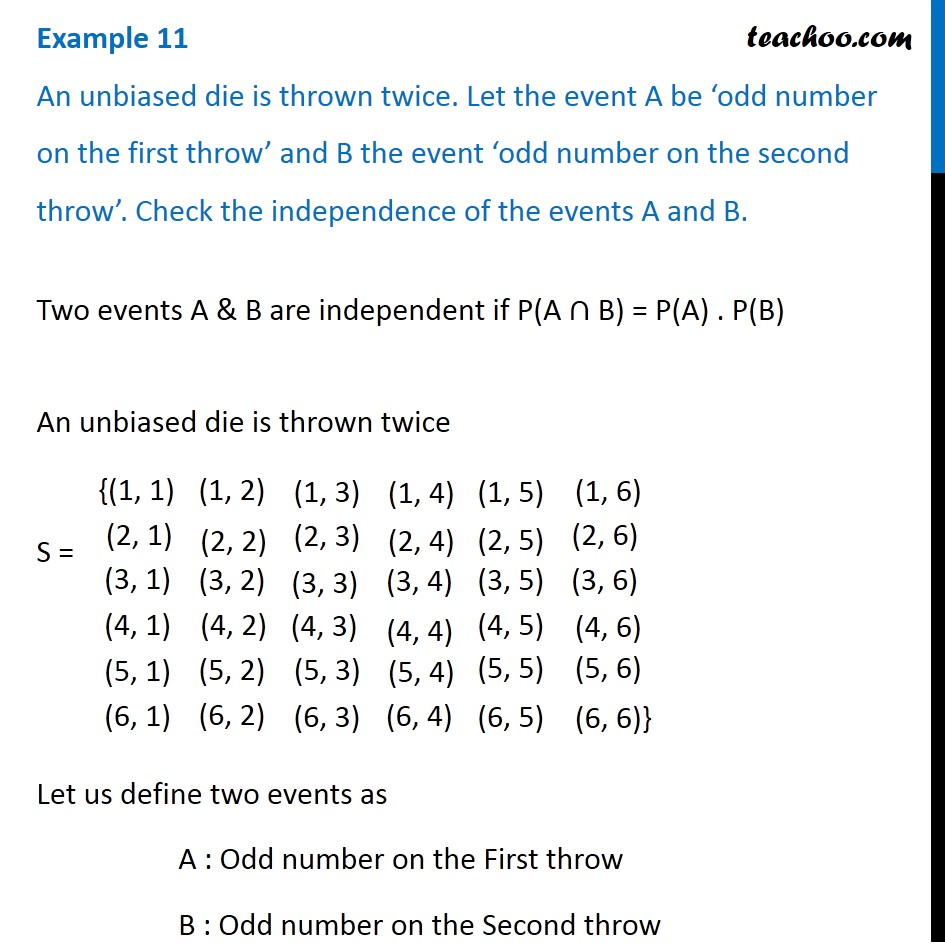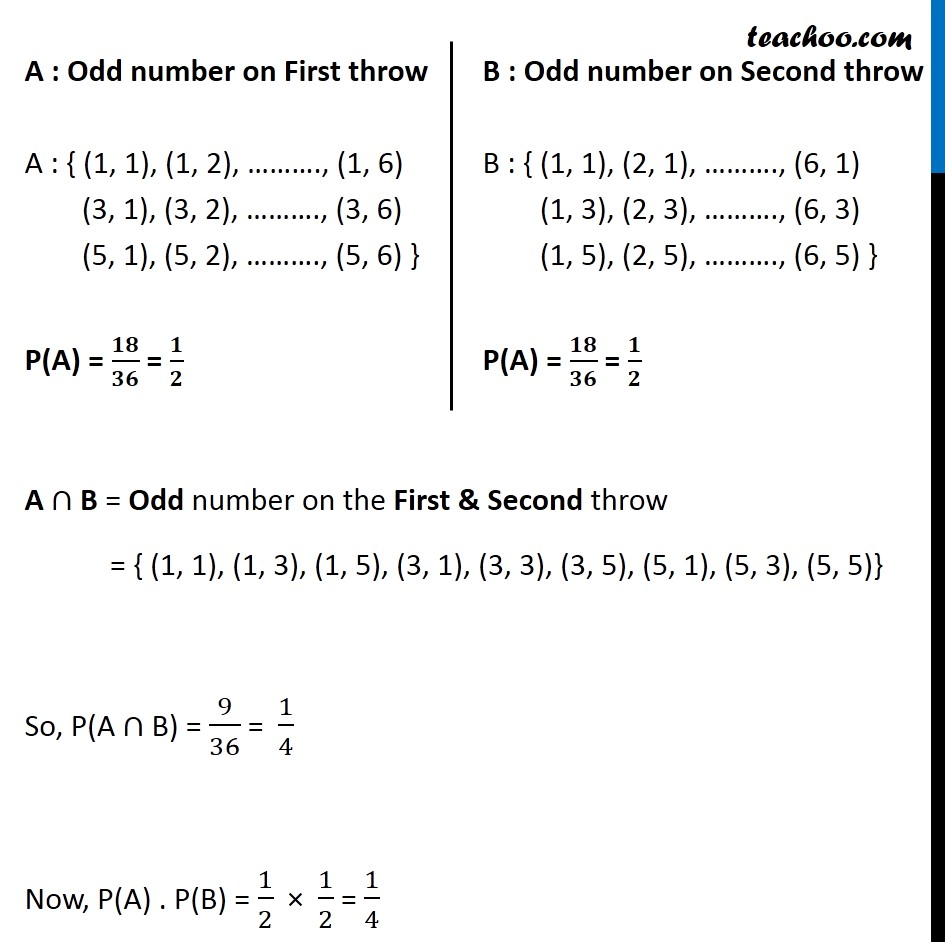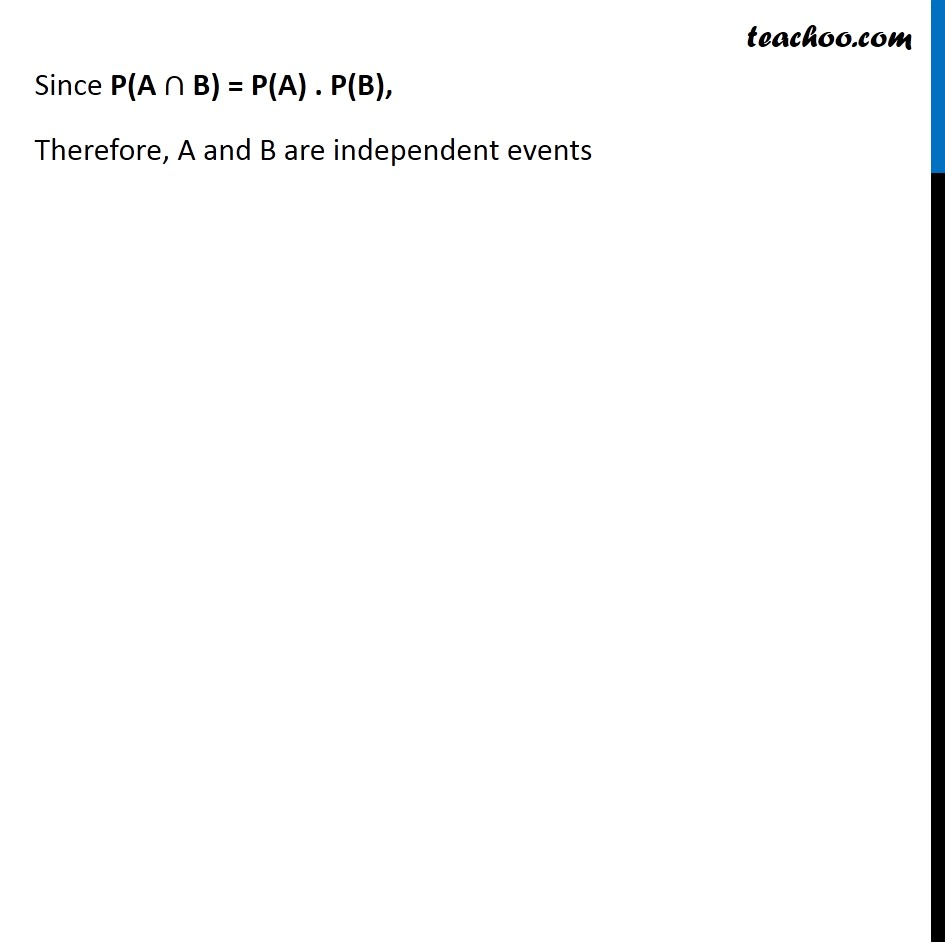Chapter 13 Class 12 Probability

Class 12
Important Questions for exams Class 12Learn in your speed, with individual attention - Teachoo Maths 1-on-1 Class

### Transcript

Example 11 An unbiased die is thrown twice. Let the event A be ‘odd number on the first throw’ and B the event ‘odd number on the second throw’. Check the independence of the events A and B. Two events A & B are independent if P(A ∩ B) = P(A) . P(B) An unbiased die is thrown twice S = Let us define two events as A : Odd number on the First throw B : Odd number on the Second throw vA : Odd number on First throw A : { (1, 1), (1, 2), ………., (1, 6) (3, 1), (3, 2), ………., (3, 6) (5, 1), (5, 2), ………., (5, 6) } P(A) = 𝟏𝟖/𝟑𝟔 = 𝟏/𝟐 B : Odd number on Second throw B : { (1, 1), (2, 1), ………., (6, 1) (1, 3), (2, 3), ………., (6, 3) (1, 5), (2, 5), ………., (6, 5) } P(A) = 𝟏𝟖/𝟑𝟔 = 𝟏/𝟐 B : Odd number on Second throw B : { (1, 1), (2, 1), ………., (6, 1) (1, 3), (2, 3), ………., (6, 3) (1, 5), (2, 5), ………., (6, 5) } P(A) = 𝟏𝟖/𝟑𝟔 = 𝟏/𝟐 B : Odd number on Second throw B : { (1, 1), (2, 1), ………., (6, 1) (1, 3), (2, 3), ………., (6, 3) (1, 5), (2, 5), ………., (6, 5) } P(A) = 𝟏𝟖/𝟑𝟔 = 𝟏/𝟐 A ∩ B = Odd number on the First & Second throw = { (1, 1), (1, 3), (1, 5), (3, 1), (3, 3), (3, 5), (5, 1), (5, 3), (5, 5)} So, P(A ∩ B) = 9/36 = 1/4 Now, P(A) . P(B) = 1/2 × 1/2 = 1/4 Since P(A ∩ B) = P(A) . P(B), Therefoare, A and B are independent events